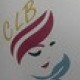Let’s do what Codea is good at – animation. We’ll make a ball fly around the screen and bounce off the sides.

First, clear out all the code in your project so you just have two empty functions like this

```function setup()
end
function draw()
end```

Drawing the ball
There are two ways to draw a ball on the screen – draw it using a prepared image, or just draw it with Codea’s tools. We’ll have a simple ball, so we’ll just draw it.

So in the draw function, we put this code

```function draw()
background(200,200,200,255) -- light gray
pushStyle(). -- remember the color/style settings
fill(255,0,0,255) --change the fill color to red
ellipse(50,50,60) --draw a circle at 50,50 with diameter 60
popStyle() --put the style settings back the way they were
end
```

Why do we draw a circle using “ellipse”? Because a circle is just a round ellipse, so one function can do both. If you want to draw a non circular ellipse you would include a fourth parameter for the height.

So when we run this, we get a red ball that just sits there.

Moving the ball
Let’s get it moving very simply. We’ll move it two pixels to the right, and one up, every time we draw. So we need to store the x and y values somewhere, starting them at 50,50, and increasing them all the time.

The best place to set the initial x,y values is in setup, because it runs first. So put this in setup..

```function setup()
x=50 --starting x
y=50 --starting y
d=60 --diameter of ball
dx=2 --the amount x changes
dy=1 --the amount y changes
end
```

Note it’s really good practice to put all our values into variables like x,y,r etc, rather than hard coding them everywhere, because it makes it very easy to change them later if we want to.

Now we need to draw the ball and make it move, like so, in the draw function.

```function draw()
background(200,200,200,255)
pushStyle()
fill(255,0,0,255)
x=x+dx -- update x
y=y+dy -- update y
ellipse(x,y,d) -- draw the ball at the new position
popStyle()
end```

If you run it, you’ll see we have a ball that moves slowly across the screen – and off the edge. We want it to bounce back. In fact this is fairly simple, if you think about it carefully.

Firstly, the x and y values are at the centre of the ball. This means the ball will touch the wall if it gets closer than its radius (which is d/2 in our example). So that’s how we test for a collision with the side.

Secondly, we need to reverse the ball direction, and this simply means changing the sign of dx and dy. So the draw function becomes this..

```function draw()
background(200,200,200,255)
pushStyle()
fill(255,0,0,255)
x=x+dx
y=y+dy
ellipse(x,y,d)
popStyle()
--change direction if we hit a wall
if x>WIDTH-d/2 or x<d/2 then dx=-dx end
if y>HEIGHT-d/2 or y<d/2 then dy=-dy end
end
```

If you run this, you’ll see we have a bouncing ball.

Here is the final code:

```function setup()
x=50 --starting x
y=50 --starting y
d=60 --diameter of ball
dx=2 --the amount x changes
dy=1 --the amount y changes
end

function draw()
background(200,200,200,255)
pushStyle()
fill(255,0,0,255)
x=x+dx
y=y+dy
ellipse(x,y,d)
popStyle()
--change direction if we hit a wall
if x>WIDTH-d/2 or x<d/2 then dx=-dx end
if y>HEIGHT-d/2 or y<d/2 then dy=-dy end
end
```

WordPress tends to mangle some of the code, so you might find it easier to copy from here.

From → animation

1.hey i have tried to copy the code exactly but each time it runs it comes up and says error: [string “function setup()…”] :12: attempt to index a nil value

•Hi clay. You’ll say I say at the end that WordPress messes up the code, so I’ve given you an external link to copy from. Use that instead.

•I found out where the error is. The “.” after the pushStyle() is what gives Clay’s error, as I removed it and it ran perfectly. Just FYI. Thanks for the tutorials!

2.i am a complete novice but i am creating my website with wpress and hostgator and want to know what you call moving objects? i would like to have in the header area sparking crystal’s or butterfies etc that flash on and off,but i don’t know what you would call this,it’s definately not called flash images which would be what i would think they are,your help is most appeciated,thank you Andrea

•You need “animated gifs”

If you google for this, you may find what you want

•thank you so much for your quick reply,am already into gifs.net and this is exactly what i wanted,now hope i can intall it correctly,but like learning how to do a website from the backend with hours and days of watching ytube leasson’s will do the same with this,or ist i might go to plugins and see what comes up in the search bar for animated gifs. Regards Andrea